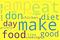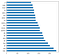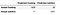# Natural Language Processing, APIs, and Classification in Python, A Project WalkthroughMost Frequent Words in Both Subreddits

The mission: create a model that distinguishes well between cooking and nutrition posts on Reddit; a natural language processing classifier problem.
Performance metrics: accuracy, and precision.
The data collection method: Reddit API through pushshift.io (resources below). I collected 8403 posts, 4166 nutrition, 4237 cooking, from 60 days before till the day of the data collection.
Vectorizers used: CountVectorizer, TfidfVectorizer to create the sparse matrix of features count/frequency respectively, to feed it to the classification model; tokenizer is included in these vectorizers.
Models used/tested: Logistic Regression, Multinomial Naive Bayes, Random Forest.
Modeling tools used: Pipelines, and GridSearch.
Evaluation methods: accuracy score, cross-validation, precision from classification report, confusion matrix to see False Positives and False Negative, ROC curve to visualize model performance.

Read on for explanation of code for each part! The full code with output is found in the Jupyter Notebook in this link https://github.com/hayatoumy/classification_api/blob/master/project_3.ipynb

Libraries Import:

`import requestsimport jsonimport pandas as pdfrom time import sleepfrom sklearn.feature_extraction.text import CountVectorizer, TfidfVectorizer, ENGLISH_STOP_WORDSfrom sklearn.linear_model import LogisticRegressionfrom sklearn.naive_bayes import MultinomialNBfrom sklearn.model_selection import GridSearchCVfrom sklearn.pipeline import Pipelinefrom sklearn.model_selection import train_test_split, cross_val_scorefrom sklearn.metrics import confusion_matrix, classification_report, accuracy_scorefrom sklearn.ensemble import RandomForestClassifier`

Collecting a 100 posts from 60 days ago till now, in reverse order:

`base_url_cook = 'https://api.pushshift.io/reddit/search/submission/?subreddit=cooking&size=100&before={}d'urls_cook = [base_url_cook.format(i) for i in range(60,-1,-1)] # generate the urls# the first -1 is the stopping point, coz range is exclusive to the endpoint. # the second -1 is to go in reverse on the range.base_url_nut = 'https://api.pushshift.io/reddit/search/submission/?subreddit=nutrition&size=100&before={}d'urls_nut = [base_url_nut.format(i) for i in range(60,-1,-1)]`

This last line is to loop through the urls, changing the parameter of how many days to go back. Thus, creating 61 links, including day 0, the current day. A cool hack using `.format()`.
Now, requesting the pages. Be sure to sleep! This is really important! So you don’t shut down the webpage’s server, or be blocked from requesting the API. Read the API documentation to know how long you need to sleep, they usually put it there, if you didn’t find any, sleep for one second at least.

`pages_cook = []for u in urls_cook:    sleep(1)    pages_cook.append(requests.get(u).json()['data'])`

And we do the same for the nutrition, I called it `pages_nut`.

`for p in pages_nut:    count2 = 0    for post in p:        try: #because one post doesn't have a 'selftext'. nut_data stops at 116. therefore I need try/except            if post['selftext']!='':                nut_data.append(post['selftext'])                nut_target.append(post['subreddit'])        except:             nut_data.append('[removed]') # i want to add what I want to drop later on. some posts have '[removed]' in them            nut_target.append('nutrition')`

I’m doing the try/except because post 117 didn’t have a ‘selftext’ tag, which gave an error, and cut the string at 116. So I put the except as ‘[removed]’ because there are removed posts filled with ‘[removed]’ that I intend to drop them later from the data frame, so I lumped them all together.
I repeated the same thing for cooking.

Making the data frame for each topic using this:

`nut = pd.DataFrame(zip(nut_data, nut_target), columns = ['post', 'topic'])`

`zip()` is my favorite tool, I used it to keep the post attached to its topic. You can zip any number of columns.

Now to zero-in on the ‘[removed]’ posts, I used `.loc[]`, got the indices of these rows, then fed it to `.drop()`; saving changes to the data frame with the `inplace = True` argument.

`nut.loc[nut['post']=='[removed]',:].indexnut.drop(nut.loc[nut['post']=='[removed]',:].index, inplace = True)`

Now I combined the two data frames for each topic into one, and saved it to a .csv file

`#combining the dataframesdf = pd.concat([cook, nut], axis = 0, sort = False)# saving it to a csv filedf.to_csv('reddit_cook_nut.csv', index = False)`

The argument `index = False` in the `to_csv()` prevents adding another index column to the dataset, usually called ‘Unnamed:0’ when you later load the csv.

Now we’re ready for some EDA (exploratory data analysis). I already know my dataset is full, because I made it to remove all empty posts.

Checking the classes count, essential first step in any classification problem, you want your classes to be balanced, i.e. same count, or close enough.
This is also an essential step to know your baseline accuracy, that is without any model what is the percentage of each class such that if I pulled a sample at random, what is the percentage of the desired class I would get

`df['topic'].value_counts() # classes size`

Here ‘topic’ is my target variable.

`df['topic'].value_counts(normalize = True) # baseline accuracy`

Will give you percentages, i.e. probabilities of each class. From there, our goal is to create a model that gives much better accuracy than the baseline accuracy. Since our classes are fairly balanced, a great thing to have, we need a model that does a lot better than 50% otherwise the posts aren’t really distinguishable from the words that appear in them.

# Stop Words

There’s a custom list of most repeated words that don’t carry meaning to our NLP analysis, such as ‘there’, ‘I’, ‘am’, ‘this’… etc. You can either add them as an a parameter in your vectorizer `stop_words = 'english'` or you can import this list from sklearn, and add to it `from sklearn.feature_extraction.text import ENGLISH_STOP_WORDS`. I did the latter, because I saw there’s other irrelevant words that showed up most in posts, like ‘know’, ‘want’, ‘just’, and some html residue. I combined the two lists like so, and called them custom_words

`more_stop = ['just', 'com', 'https', 'know', 'want', 'www', 've','x200b', 'really', 'like'] custom_words = list(ENGLISH_STOP_WORDS) + more_stop`

Now I fed that to my vectorizer, and created the sparse matrix of word counts:

`cv = CountVectorizer(stop_words = custom_words)sparse_mat = cv.fit_transform(df['post']) #fitting the model`

In order to plot the most repeated words, I need the column names from the sparse matrix. However, by definition, a sparse matrix is an array of points to values, not actual values. To circumvent this, we need to change it to a regular matrix (a dense matrix); then extract column names. CountVectorizer has an included method `.get_feature_names()` that gets the names for us. I put all that in a data frame to use it later in plotting. All together, this is one line of code:

`all_feature_df = pd.DataFrame(sparse_mat.todense(), columns=cv.get_feature_names()) #attaching column names i.e. words`

Now I can simply do:

`all_feature_df.sum().sort_values(ascending=False).head(20).plot(kind='barh');`

Recall we have a matrix of word counts in each post (row). This last line of code says: sum up all columns, sort them from highest to lowest sum, give me only the first 20 of these, and plot them in a horizontal bar plot. The result is an easy to understand visual,Most Repeated Words in Both Topics’ Posts

Keep in mind, what you see is lemmatized words, i.e. truncated to, hopefully, the stem of the word, but lemmatization and stemming are not accurate enough methods. So, most likely “don” was “don’t” but I’m not sure, so I didn’t take it out by adding it to the list of stop words.
By category, the most frequent words appeared this many times

The code that generates these last graphs:

`common_words_indicies = all_feature_df.sum().sort_values(ascending=False).head(20).index# we need the indices because that's what .loc is looking for (or a boolean mask) when I use it belowdf_nutrition = sparse.loc[df['topic'] == 'nutrition'].copy()df_cooking = sparse.loc[df['topic'] == 'Cooking'].copy()fig, ax = plt.subplots(nrows = 1, ncols = 2, figsize = (10,8), sharex=True) #last argument to set same scale for xdf_nutrition.sum().loc[common_words_indicies].plot(kind = 'barh',ax = ax, title = 'common words in relation to Nutrition posts'.title());df_cooking.sum().loc[common_words_indicies].plot(kind = 'barh', ax= ax, title = 'common words in relation to cooking posts'.title());`

I used `.copy()` to create a physical data frame rather than a pointer of the original. Thus, when I make changes to the sub-dataframes, the original wouldn’t change. Python creates only pointers to the original data frame to save storage and memory.

# Selecting and Evaluating Models:

I used both cross-validation, and train/test/split to evaluate my models performance for 20% of data as test data. I have large enough dataset to do this.
I will also combine pre-processing (vectorizing), and modeling in pipelines to make things easier to track and use GridSearch on to fine-tune parameters. I used combinations of TF-DIF vectorizer, and CountVectorizer with Naive multinomial Bayes, and Logistic regression. I also used random forests, but it did the worse with 91% accuracy.
The winning model was the TFDIF with Logistic Regression with 98% accuracy score. Here’s the pipeline and the GridSearch

`tf_log_pipe = Pipeline([('tfdf', TfidfVectorizer()),('logreg', LogisticRegression())])tf_log_params = {    'tfdf__stop_words' : [custom_words, None],    'logreg__penalty' : ['l1', 'l2'],    'logreg__C' : [.1, 5, 50]}tf_log_gs = GridSearchCV(tf_log_pipe, tf_log_params, cv = 5, verbose=0)tf_log_gs.fit(X_train, y_train)print('best score of TFDIF with Logistic Regression is:', tf_log_gs.best_score_.round(4))`

I then used `print(tf_log_gs.best_estimator_.get_params()['steps'])` to see these best parameters and use them to fit this model to the training data, and predict on the testing data.
Note that `best_params()` gives a dictionary, with a key in it names `steps` which contains the parameters I’m looking for, for the vectorizer and the logistic regression model.

`tf = TfidfVectorizer(stop_words = custom_words)X_train_tf = tf.fit_transform(X_train)X_test_tf = tf.transform(X_test)print(X_train_tf.shape)logreg = LogisticRegression(C = 50, penalty = 'l2') #l2 is Ridge logreg.fit(X_train_tf, y_train)preds = logreg.predict(X_test_tf)`

After that, I found the confusion matrix, to check our the False Positives and False Negative counts. Checked the classification report for more metrics like precision and f1-score.

`confusion_matrix(y_test, preds)`

# Plotting Coefficients

I did this to see the most impactful words. That is, words that best separate posts and classify them as “cooking” vs “nutrition”.
Get the coefficients: `tf_log_gs.best_estimator_.steps.coef_ # same as logreg.coef_`
Making coefficients into a data frame to plot them.

`coef_df = pd.DataFrame(logreg.coef_, columns = tf.get_feature_names()).T.sort_values(by = 0).head(15)coef_df['abs'] = coef_df.abs()coef_df.sort_values(by = 'abs', ascending = False).head(15)`

Plotting most important (strongest) coefficients:

`coef_df.sort_values(by = 'abs', ascending = False).head(15).plot(kind = 'barh', figsize = (10,10), title = "most \important 15 coefficeints in our model, with respect to class: nutrition".title(), fontsize = 15);`

Notice how all are negative? That means that existence of these words is the strongest indication the post comes from Cooking (not Nutrition). Remember, SKlearn thinks class zero is whatever comes alphabetically first between the two, unless you manually coded that otherwise early on.
Interpretation for the ‘make’ coefficient: one unit increase in the frequency of the word ‘make’ appearance in a post, reduces the probability of being in Nutrition by approximately 0.00029; holding all the other coefficients constant. Or equivalently, increases the probability of being in Cooking by 0.00029 holding all the other variables constant.

# Raising the Threshold

It’s straightforward to compute the confusion matrix in SKlearn, just remember negative comes before positive class. Also, columns are predictions, rows are actuals.

`preds = logreg.predict(X_test_tf)#logreg is the logistic regression model with TFDIF vectorizercol_names = ['Predicted ' + i for i in df['topic'].value_counts().index]index_names = ['Actual ' + i for i in df['topic'].value_counts().index]cm = pd.DataFrame(confusion_matrix(y_test, preds), columns = col_names, index = index_names )`Confusion Matrix For The Two Classes

The default threshold is 0.5, more than that is classified in class 1, less in 0. However, if you would like to minimize False Negatives or False Positives, you want to decrease or increase the threshold, respectively.
You’ll have to do that manually, like so:

`# getting prediction probabilities from our winning model, only for the positive class probs_nut = tf_log_gs.predict_proba(X_test)[:,1]# setting the threshold, and getting the new predictions/classifications: def classify(thresh, probs_list):    """    thresh: threshold of classification     probs_list: a list of predict_proba of only the class of interest. Must be worked outside the function     """    preds_thresh = ['nutrition' if probs_list[i] >= thresh else 'Cooking' for i in range(len(probs_list))]    return preds_thresh# getting the confusion matrix with the new threshold predictions, printed nicely:  pd.DataFrame(confusion_matrix(y_test, classify(0.9, probs_nut)), columns = col_names, index = index_names )# col_names, and index_names are defined above`

I decided against changing the threshold in this context, because there’s no particular risk of more False Positives vs False Negatives; also, I wanted to have a balance between two types of error. Because every time you minimize one, the other error will go up. In some cases you do want to minimize False Negatives though even at the cost of increasing False Positives; like when you’re dealing with cancer screening, or fraudulent transactions; because you don’t want to send home a sick patient, or let a fraudulent transaction slip.

# ROC curve:

One last piece to visualize your classification model performance: use the ROC curve, you want the blue curve to be as close as possible to a square corner, thus making the area under the curve as close to 1 as possible; to visualize model performance over all possible thresholds at once:

`# Finally, visualizing our model performance, using ROC curve:from sklearn.metrics import roc_curve# to set up for ROC curve y_numerical = y_test.map({'nutrition' : 1 , 'Cooking' : 0})fpr, tpr, _ = roc_curve(y_numerical, probs_nut)plt.figure(figsize = (6,6))plt.plot(fpr, tpr);plt.plot([0,max(y_numerical)],[0, max(y_numerical)], '--'); # it takes only encoded numerical yplt.title('ROC curve for the TFDIF vectorizer with logistic regression'.title());plt.xlabel('false positive rate'.title());plt.ylabel('true positive rate'.title());`

My model did really well here as you see, I had 98% accuracy on testing set.

You could have built the ROC curve manually by computing the FPR and FNR:
False positive rate = type I error =1 − specificity = FP / (FP + TN)
False negative rate = type II error = 1 − sensitivity = FN / (TP + FN)

NLP seems a lot to take in at first, but shortly after you’ll be comfortable with it. I hope I could show you a complete example from collecting data to inference.

I welcome feedback and sharing thoughts!

# Resources:

There are many sources out there explaining count vectorizers, TF-DIF frequency vectorizers, ROC curve, confusion matrix, Type I and Type II errors; pick what you like, I can’t recommend just one.

API documentation: GitHub — pushshift/api: Pushshift API
subreddits list: reddit: the front page of the internet
You will find the links I used, and the full Jupyter Notebook on GitHub repository (insert this repo link here).
My portfolio: Hello and Welcome! | Haya Toumy